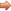# JavaScript & jQuery Tutorials

JS HomeJS ReferenceBitwise

## Bitwise operators

Used for 32 bit manipulation.

### Description

Bitwise operators perform their operations by processing their operands as a sequence of 32 bit binary integers (zeros and ones). The bit with the lowest value is placed on the right and non values are zero filled. After the processing is completed the operands are converted back to standard Javascript numerical values before returning them.

The following shows the value of the right most 8 bits and some values so you get an idea of binary notation.

bit8bit7bit6bit5bit4bit3bit2bit1 Decimal Value
000000011
000000113
000001117
0000111115
0001111131
0011111163
01111111127
11111111255

In the examples below we are just showing the first 8 bits of the 32 bits where possible as it makes the examples easier to understand.

## Bitwise Logical Operators

When we have two operands in bitwise logical operators, each operand is converted to 32 bit binary integers. Once this is done corresponding bits in each operand are tested against the logical operator and the result is constructed bit by bit, before being converted back to standard Javascript numerical values.

### `&` - Bitwise AND

This operator performs the AND operation on the corresponding pair of bits in each operand and only returns a 1 when both bits = 1.

Lets see an example and how we get to the answer.

``````
// Function context.
var a = 247, b = 207;
c = a & b;

``````

Press the button below to action the above code:

Lets see how we got 199 as an answer

Decimal Binary Result
247 `11110111`
207 `11001111`
`--------`
`11000111` 199

### `|` - Bitwise OR

This operator performs the OR operation on the corresponding pair of bits in each operand and returns a 1 when either bit = 1.

Lets see an example and how we get to the answer.

``````
// Function context.
var a = 247, b = 207;
c = a | b;

``````

Press the button below to action the above code:

Lets see how we got 255 as an answer

Decimal Binary Result
247 `11110111`
207 `11001111`
`--------`
`11111111` 255

### `^` - Bitwise XOR

This operator performs the XOR operation on the corresponding pair of bits in each operand and returns a 1 when the bits are different.

Lets see an example and how we get to the answer.

``````
// Function context.
var a = 247, b = 207;
c = a ^ b;

``````

Press the button below to action the above code:

Lets see how we got 56 as an answer

Decimal Binary Result
247 `11110111`
207 `11001111`
`--------`
`00111000` 56

### `~` - Bitwise NOT

This operator performs the NOT operation on each bit inverting the value (one's complement).

Lets see an example and how we get to the answer.

``````
// Function context.
var a = 247;
b = ~a;

``````

Press the button below to action the above code:

Lets see how we got -248 as an answer

Decimal Binary Result
247 `00000000000000000000000011110111`
`--------------------------------`
~ `11111111111111111111111100001000` -248

## Bitwise Shift Operators

The bitwise shift operators take two operands, the first being the value to be shited and the second the number of bits to shift the value by. The shift direction is dependant upon the shift operator used. Results are returned with the same type as the left operand.

• The right operand value should be less than 32, so only the rightmost 5 bits will be used in calculations and the rest ignored.

### `<<` - Bitwise Left Shift

The bitwise left shift operator shifts the left operand to the left by the number of bits specified by the right operand.

• Any bits shifted off to the left past the 32 bit boundary are discarded.
• Zero bits are shifted in from the right.

Lets see an example and how we get to the answer.

``````
// Function context.
var a = 15;
b = a << 3;

``````

Press the button below to action the above code:

Lets see how we got 120 as an answer

Decimal Shift bits 3 to left After Shift Result
15 `00001111` `01111000` 120

### `>>` - Bitwise Sign-propagating Right Shift

The bitwise sign-propagating right shift operator shifts the left operand to the right by the number of bits specified by the right operand.

• Any bits shifted off to the right past the 32 bit boundary are discarded.
• Copies of the leftmost bit (the sign bit) are shifted in from the left so the sign of the left operand is preserved (propogated).

Lets see an example and how we get to the answer.

``````
// Function context.
var a = 76;
b = a >> 3;

``````

Press the button below to action the above code:

Lets see how we got 9 as an answer

Decimal Shift bits 3 to right After Shift Result
15 `01001100` `00001001` 9

### `>>>` - Zero-fill Right Shift

The bitwise zero-fill right shift operator shifts the left operand to the right by the number of bits specified by the right operand.

• Any bits shifted off to the right past the 32 bit boundary are discarded.
• Zero bits are shifted in from the left, so the result will always be positive.
• For positive numbers results are always the same at the self-propogating right shift operator.
• For negative numbers the left most bit (the sign bit) is shifted to the right instead of being copied so results will return large numbers.

Lets see an example and how we get to the answer.

``````
// Function context.
var a = 76;
b = a >>> 3;

``````

Press the button below to action the above code:

Lets see how we got 9 as an answer

Decimal Shift bits 3 to right After Shift Result
15 `01001100` `00001001` 9

## Related Tutorials

JavaScript Basic Tutorials - Lesson 5 - Basic Maths Functions